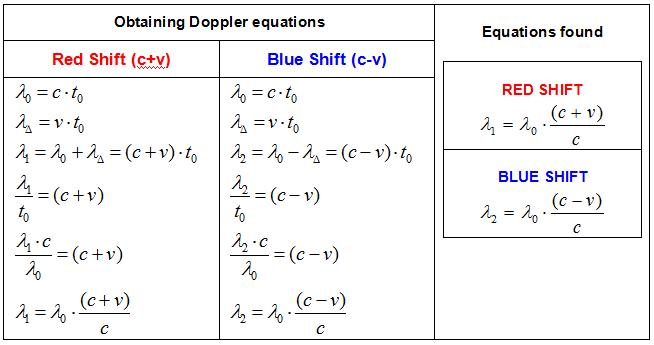26. EMERGENCE OF DOPPLER SHIFT EQUATIONS DURING THE EMISSION OF AN ELECTROMAGNETIC WAVENow that Doppler Shift occurs at the moment of signal emission and at the source, then lets see this occurrence on a graphics first and then see whether Doppler Shift equations emerged mathematically or not.Lets assume that the factory setting of the signal transmitter is f0 for the frequency and λ0 for the wavelength. Lets discuss a piece of the signal that set out from the transmitter, which is one wavelength long. Since the signals went to three different targets, the wavelengths of these signals that went to three targets were formed differently. On the other hand, although the wavelengths of the signals that are emitted, the fact that the frequency doesnt change means that the durations of the emissions of the signals over λ0, λ1, λ2 wavelengths are equal. We can get the duration of the wavelength emission from the factory settings of the transmitter. This duration is λ0/ c = t0. When we multiply the difference between the reference systems that lead to the formation of Doppler, namely v, with the duration of the wavelength emission t0, we find the amount that leads to the change in the wavelengths of the signals that go to targets that are in motion.On the other hand, the wavelength is a physical quantity, a length, a distance. Even though their lengths are different, emission of signal wavelengths that go to three different targets in the same duration of time directly gives the speeds of the signals that go to targets that are in motion, as well. And this is clear information.Since;The speed of the signal that goes to the plane that is moving away =The speed of the signal that goes to the plane that is approaching =Consequently, we get: c1 > c > c2Lets see if we can get Doppler equations by making use of the figure above. We can do it easily as can be seen below.As we mentioned before, the terms Red Shift and Blue Shift in Doppler Shift are used to express the increase and decrease in the energy of the electromagnetic wave. Red Shift is lengthening of the wavelength of the electromagnetic wave; it indicates a decrease in the energy and is represented by (c+v). Blue Shift is shortening of the wavelength of the electromagnetic wave; it indicates an increase in the energy and is represented by (c-v).